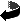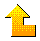Statistics 679: Time Series Analysis II

 Professor Douglas Wiens Office: CAB 429 Email: doug.wiens@ualberta.ca Reload to change picture

## Course materials:

• Course information, assessment procedures, etc.
• Lecture notes. These are constantly under revision, before and after class.
• The course assumes an undergraduate knowledge of Time Series at the level of STAT 479, and mathematics at the level of STAT 512.

## Obtaining and using the R package:

• For a quick start you can obtain R here and install it on a PC by double-clicking on this .exe file and following the instructions. There is a Mac version of R too; you'll need to consult the 'R home page' link below for this.
• There is a problem with R version 2.8 which impacts us - see the notes by S&S.  But version 2.7 is fine and can be obtained here.

• Typographical errors in the text.
• Some further (and very useful) information about R; see in particular the authors' R tutorial and some of their caveats regarding R for time series analysis.
• Datasets used in the text.  These can be saved to your own system individually, or you can download all of them at once as data.zip.
• Some R functions supplied by Shumway & Stoffer and extended and modified by me. See the notes by S&S. You can make their (unmodified) functions available by entering a command source("http://www.stat.pitt.edu/stoffer/tsa2/Rcode/itall.R") within any R programme.
• Further R functions and code unique to Chapter 6.  You can make these functions available by entering a command source("http://www.stat.pitt.edu/stoffer/tsa2/Rcode/New6/ssall.R") within an R programme.
• Code unique to Chapter 7.

## Technical Writing:

• The importance of clarity of exposition, and of grammatical correctness, in technical writing cannot be over-emphasized. The best way to determine if you understand what you are doing is to try to write it down in a form that another reader can understand. Some comments along these lines are included with the course information; for some helpful resources see "Writing Aids" on my homepage.

## Code for R examples discussed in class (some of these originated at the authors' website): You should find it useful to download and run these R scripts before the classes in which they are to be discussed.

• Lecture 1 - SOI and Recruits; ACF, CCF, regression.
• Lecture 2 - Varve series; estimation and forecasting (Example 3.31).
• Lecture 4a - SOI and Recruits; spectrum estimation, coherence (Examples 4.11, 4.16).
• Lecture 4b - SOI and Recruits; impulse-response; optimal filtering (Examples 4.23, 4.24).
• Lecture 5 - Varve; long memory (Example 5.1).
• Lecture 6a - NYSE; GARCH fit (Example 5.4).
• Lecture 6b - Flu; threshold modelling (Example 5.5).
• Lecture 7a - Mortality; regression modelling (Example 5.6).
• Lecture 7b - SOI and Recruits; transfer functions (Example 5.8).
• Lecture 9 - VAR modelling of Mortality data (Examples 5.9, 5.10, 5.11).
• Lecture 12 - Kalman filter and smoother on simulated data (Example 6.5).
• Lecture 13a - Global Temperature series; ML estimation (Example 6.7).
• Lecture 13b - Simulated data; ML estimation via EM algorithm (Example 6.8).
• Lecture 14 - J&J Quarterly Earnings; structural modelling (Example 6.10).
• Lecture 15 - Newbold data; bootstrapping (Example 6.12).
• Lecture 19 - Shasta Lake data; multiple regression in frequency domain (Example 7.1); calls stoch.reg.R.
• Lecture 20 - "Nuke" data; regression with deterministic inputs (Example 7.4).  Contributed by Fraser Newton.
• Lecture 21 - "Nuke" data; regression with random coefficients (Example 7.6).  Contributed by Junfeng Ma.
• Lecture 22a - fMRI data (all 234 series) arranged so as to produce Figure 22.1.
• Lecture 22b - fMRI anova (Examples 7.7, 7.8).  Contributed by James Ng.
• Lecture 23 - Principal component analysis of fMRI data (Example 7.14).
• Lectures 24a - Discriminating earthquakes from explosions in time domain (Example 7.11).
• Lecture 24b - Discriminating earthquakes from explosions in frequency domain (Example 7.12).
• Lecture 25 - Clustering earthquakes and explosions (Example 7.13).

## Statistics resources at U of A:Homepage of Doug WiensHomepage of Robert ShumwayHomepage of David StofferDepartment of Mathematical and Statistical Sciences Home Page